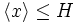# Variety-containing implies omega subgroup in group of prime power order

(diff) ← Older revision | Latest revision (diff) | Newer revision → (diff)

## Definition

Suppose$P$ is a group of prime power order, i.e., a finite$p$-group for some prime number$p$. Suppose$H$ is a Subisomorph-containing subgroup (?) of$P$: a subgroup of$P$ such that any subgroup of$P$ isomorphic to a subgroup of$H$ is itself contained in$H$. Then,$H$ is one of the omega subgroups of$P$. In other words, there is some$k$ such that:$H = \Omega_k(P) := \langle x \mid x^{p^k} = e \rangle$.

## Proof

Given: A finite$p$-group$P$, a subisomorph-containing subgroup$H$ of$P$.

To prove:$H = \Omega_k(P)$ for some natural number$k$.

Proof: Let$p^k$ be the exponent of$H$. Then, we clearly have:$H \le \Omega_k(P) = \langle x \mid x^{p^k} = e \rangle$.

Next, we show that$H = \Omega_k(P)$. Suppose$x \in P$ is such that$x^{p^k} = e$. Then, since$p^k$ is the exponent of$H$, there exists$y \in H$ such that the order of$y$ is$p^k$. Suppose the order of$x$ is$p^l$,$l \le k$. Then,$\langle x \rangle \cong \langle y^{p^{k-l}} \rangle$. Thus,$\langle x \rangle$ is isomorphic to a subgroup of$H$, so$\langle x \rangle \le H$. Thus,$\Omega_k(P) = H$.